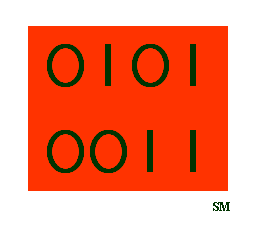Ersatz Systems Machine Cognition, LLC
Let the bits speak for themselves.
Products
Bivalent Neuron BVNsm
A special Kanban cell using bivalent logical connectives maps the neuron. This uses Logical Connective Implementation (LCI) below. See the Downloads section for documentation.
Kanban Cells
The word Kanban means card in Japanese. It was originally used to describe automobile models to be built on a certain date so that the inventory of components would be exact. An abstraction of the Kanban cell describes many things such as accounting arithmetic in a generic N-entry bookkeeping system. The Kanban cell also describes the neuron.
Logical Connectives
Logical connectives perform a specific function on two terms such as the precedent and antecedent in a mathematical expression. In computer science, connectives are known as the operators AND, OR, XOR. Logical connectives drive the BVN through the Kanban cell model. The method is known as Logical Connective Implementation (LCI).
φΦ Phigit Computing
The Greek letter phi is the golden ratio of ( 1 ± ( 5 ^ 0.5)) / 2. By convention, lower case phi φ is the negative solution, and upper case phi Φ is the positive solution, with the unique property of Φ = 1/φ. Phi appears throughout the natural world, and produces appealing proportions in visual art.

Phigit computing is arithmetic in radix-phi as maximal baseΦ, or minimal baseφ, and uses the Standard Notation below.

Standard Notation

Standard notation is a method to convert radix-phi into binary powers of phi. For example, in minimal baseφ the number 5 = 1000.1001, and in maximum baseΦ the number 5 = 101.1111 . Standard notation also has certain rules such that numbers are expressed in a minimal number of 1's, 0's, or digits. Standard notation may be helpful to perform arithmetic in the BVN.